• 矩阵行列式的计算在数学上有多种方法，为了编程方便，我采取将矩阵转化为上三角的形式，然后对角线上的数相乘，便得到行列式的值。 首先将矩阵储存在vector的二维数组中，接着开始进行行与行之间的·换算。使矩阵...
1.行列式的计算

矩阵行列式的计算在数学上有多种方法，为了编程方便，我采取将矩阵转化为上三角的形式，然后对角线上的数相乘，便得到行列式的值。

首先将矩阵储存在vector的二维数组中，接着开始进行行与行之间的·换算。使矩阵转换为上三角形式。

以下是代码：

void CJZCalculate3Dlg::det()
{

vector<vector<double > > v5=v1;        //  v1是与edit框绑定的变量所表示的矩阵
vector<vector<double > > v6(100);
for(int tt=0;tt<r1;tt++)
v6[tt].resize(c1);
double c={0};
double det=1;

for(int i=0;i<c1-1;i++)    /   上三角运算
{
if(v5[i][i]==0)       /// 判断 对角数是否为 0
{
int flag=0;
for(int f=i+1;f<c1;f++)
{
if(v5[f][i]!=0)
{
v6=v5[i];
v5[i]=v5[f];
v5[f]=v6;
flag=1;
det=0-det;
break;
}
}
if(flag==0)
{
det=0;
break;
}
}     ///   结束判断

c[i]=v5[i][i];
for(int j=i+1;j<c1;j++)
v5[i][j]=v5[i][j]/v5[i][i];
v5[i][i]=1;
for(int k=i+1;k<c1;k++)
{
for(int t=i+1;t<c1;t++)
v5[k][t]=v5[k][t]-v5[k][i]*v5[i][t];
v5[k][i]=0;
}
}
det=det*v5[c1-1][c1-1];
for(int s=0;s<c1-1;s++)
det=det*c[s];

m_str3.Format(_T("%lf "),det);     ///m_str3为与edit框绑定的变量，update后便可以将行列式的值刷新到edit框

}
det的值便为行列式的值

2.逆矩阵的求导

首先，确定待求矩阵行列式是否为0，如果为0.则不存在逆矩阵

确定了存在逆矩阵后，便可以开始进行计算了；

利用数学公式：矩阵A的矩阵逆矩阵对于，A的伴随矩阵除以A的行列式。

以下为代码：

void CJZCalculate3Dlg::OnButton6()
{

///       m_str3为与edit框绑定的变量

m_str3="";  //情况edit3里的数据，以便显示新数据

det();    //  计算行列式
double A=atof(m_str3);       /   行列式赋值给A
if(A==0)
{
m_str3="行列式为0，不存在逆矩阵";
}
else
{
double a={0};

v7=v1;
c1--;
for(int i=1;i<=r1;i++)             //伴随矩阵计算
{
for(int j=1;j<=r1;j++)
{
v1.erase(v1.begin()+i-1);
for(int k=0;k<r1-1;k++)
v1[k].erase(v1[k].begin()+j-1);
for(int k2=0;k2<r1-1;k2++)
v1[k2].resize(r1-1);

det();
a[j][i]=atof(m_str3);

if((i+j)%2==1)
a[j][i]=0-a[j][i];

for(int jk=0;jk<r1;jk++)
v1[jk].resize(r1);
v1=v7;
}
}              将伴随矩阵代入数组

c1++;
m_str3="";
for(int t1=1;t1<=r1;t1++)     /// 显示逆矩阵
{
for(int t2=1;t2<=r1;t2++)
{
str3.Format(_T("%lf "),a[t1][t2]/A);
m_str3=m_str3+str3;
}
m_str3=m_str3+"\r\n";
}
}
CString类型的m_str3就是逆矩阵
UpdateData(false);      //逆矩阵被刷新到edit框上
}

矩阵计算器完整代码见https://download.csdn.net/download/cyl00cyl/10693382，能够实现矩阵加减乘运算，幂运算，数乘运算，转置，行列式，逆矩阵。 能够检测矩阵是否完整，能够识别是否能进行所选择的运算，并且提示出不能进行计算的原因。
展开全文• ## ACM矩阵行列式计算

千次阅读 2012-07-27 15:31:14
计算行列式的基本方法就是把矩阵化成上三角或下三角，然后观察对角线的元素，如果其中有个一元素为0则整体为0，否则行列式的值就是对角线上各个元素的乘积。  先说明一下行列式的几个性质：       ...
ACM行列式计算
计算行列式的基本方法就是把矩阵化成上三角或下三角，然后观察对角线的元素，如果其中有个一元素为0则整体为0，否则行列式的值就是对角线上各个元素的乘积。
先说明一下行列式的几个性质：举个例子要将此行列式转化成上三角，根据以上行列式的性质，要将2,3,4行的第一列数字转化成0时，分别要加上第一列的-2/3倍,-3/4倍,-2/3倍；
但是我们不想乘上分数，因为计算机计算的时候会造成一定的精度误差。所以我们采用另一种方式，利用最大公约数（gcd）来讲行列式装换成上三角行列式
以第一行和第二行为例
3=2*1+1   ------>  2=1*2+0
第一步：如上图前两行，第一步讲3,2根据gcd的3=2*1+1
------>   2=1*2+0
转化成1,2，此时还没有化成0，继续转化2=1*2+1
------>   1=1*1+0
讲1,2转化成1，0，转化完成；
检查变化次数，根据上面行列式的性质，如果为偶数次，行列式D=D'，如果是奇数次D=-D'
一二行的第一个数转化好之后，就转化1,3行的，一次类推
给出行列式代码以供参考：

#include<iostream>
#include<cstdio>
#include<cmath>

using namespace std;

typedef __int64 lld;

lld a;

int sign;
lld N,MOD;
void solved()
{
lld ans=1;
for(int i=0;i<N;i++)//当前行
{
for(int j=i+1;j<N;j++)//当前之后的每一行，因为每一行的当前第一个数要转化成0（想想线性代数中行列式的计算）
{
int x=i,y=j;
while(a[y][i])//利用gcd的方法，不停地进行辗转相除
{
lld t=a[x][i]/a[y][i];

for(int k=i;k<N;k++)
a[x][k]=(a[x][k]-a[y][k]*t)%MOD;

swap(x,y);
}
if(x!=i)//奇数次交换，则D=-D'整行交换
{
for(int k=0;k<N;k++)
swap(a[i][k],a[x][k]);
sign^=1;
}
}
if(a[i][i]==0)//斜对角中有一个0，则结果为0
{
cout<<0<<endl;
return ;
}

else
ans=ans*a[i][i]%MOD;

}

if(sign!=0)
ans*=-1;
if(ans<0)
ans+=MOD;
printf("%I64d\n",ans);
}
int main()
{
int t;
scanf("%d",&t);

while(t--)
{
sign=0;
scanf("%I64d%I64d",&N,&MOD);
for(int i=0;i<N;i++)
for(int j=0;j<N;j++)
scanf("%I64d",&a[i][j]);
solved();
}
return 0;
}


贴上一个题目：

Game

时间限制(普通/Java) : 5000 MS/ 10000 MS          运行内存限制 : 65536 KByte
总提交 : 23            测试通过 : 6

描述

lxhgww feels quite bored these days,so he invented some weird game to kill time."Counting is Easy" is undoubtedly the weirdest,it's played on a N*N chess board.You are supposed
to put N rooks on the chess board,and there shouldn't be two these are in the same row or column.There are many different assignment,and it's clear that the exact number is N!,so this is not your task.In this game,there is a positive integer number on each
cell of the board,an assignment's value is the product of all the numbers whose cells are occupied by rooks.To make the game more interesting,an assignment may be nagetive or possitive,the sign is decided by the number of "Z Pairs".If there is a pair of rooks
in the assignment,and one is on the right-top direction(any angle is accepted) to another,this pair is a Z Pair.If there are odd number of Z Pairs,this assignment is negative,otherwise is positive.The goal of this game is to summarize all the N! assignment's
value,don't forget to use minus operation while the assignment is negative.The final answer S is too large to write down,so your task is just to calculate S%P's value.

输入

The first line of input contains an integer T, indicate the number of test cases in the input.Each test case begins with two integer numbers N(where 2<=N<=200) and P(2<=P<=1000000000).The
next N lines each contains N integer numbers,which describes the value of each chess board cell,all the numbers are in the range of [0,1000000000].The input is terminated by an invalid test case with N = P = 0, which should not be processed.

输出

For each test case,output one single line contains the answer S%P.

样例输入

1
2 10
4 9
2 8

样例输出

4

提示

There are two assignments(# means Rook):

*#

#*

#*

*#

First assignment's value is 2*9=18,and there are one Z-Pair,so it's negative.

Second assignment's value is 4*8=32,and there are no Z-pair,so it's positive.

So the total value S= -18 + 32 =14,and S%P=14%10=4

So the total value S= -18 + 32 =14,and S%P=14%10=4

题目来源

08年四川省省赛


展开全文• 矩阵的行列式, 指正方矩阵的行列式. ...对于简单的2阶和3阶的矩阵行列式的表达式相对简单，而且恰好是每条主对角线（左上至右下）元素乘积之和减去每条副对角线（右上至左下）元素乘积之和（见图中红线和蓝线）。 ...


矩阵的行列式, 指正方矩阵的行列式.
计算方法参考 :
http://zh.wikipedia.org/wiki/%E8%A1%8C%E5%88%97%E5%BC%8F
如图 :对于简单的2阶和3阶的矩阵，行列式的表达式相对简单，而且恰好是每条主对角线（左上至右下）元素乘积之和减去每条副对角线（右上至左下）元素乘积之和（见图中红线和蓝线）。
2阶矩阵的行列式3阶矩阵的行列式在R中使用det可以迅速的得到矩阵的行列式的值.
需要注意矩阵必须是正方矩阵.> x <- matrix(1:12,3,4)
> x
[,1] [,2] [,3] [,4]
[1,]    1    4    7   10
[2,]    2    5    8   11
[3,]    3    6    9   12
> det(x)
Error in determinant.matrix(x, logarithm = TRUE, ...) :
'x' must be a square matrix
> x <- matrix(1:16,4,4)
> det(x)
 0
> x
[,1] [,2] [,3] [,4]
[1,]    1    5    9   13
[2,]    2    6   10   14
[3,]    3    7   11   15
[4,]    4    8   12   16
> det(t(x))
 4.733165e-30
> t(x)
[,1] [,2] [,3] [,4]
[1,]    1    2    3    4
[2,]    5    6    7    8
[3,]    9   10   11   12
[4,]   13   14   15   16
[参考]
1. http://zh.wikipedia.org/wiki/%E8%A1%8C%E5%88%97%E5%BC%8F
2. > help(det)det                    package:base                    R Documentation

Calculate the Determinant of a Matrix

Description:

‘det’ calculates the determinant of a matrix.  ‘determinant’ is a
generic function that returns separately the modulus of the
determinant, optionally on the logarithm scale, and the sign of
the determinant.

Usage:

det(x, ...)
determinant(x, logarithm = TRUE, ...)

Arguments:

x: numeric matrix: logical matrices are coerced to numeric.

logarithm: logical; if ‘TRUE’ (default) return the logarithm of the
modulus of the determinant.

...: Optional arguments.  At present none are used.  Previous
versions of ‘det’ allowed an optional ‘method’ argument.
This argument will be ignored but will not produce an error.

Details:

The ‘determinant’ function uses an LU decomposition and the ‘det’
function is simply a wrapper around a call to ‘determinant’.

Often, computing the determinant is _not_ what you should be doing
to solve a given problem.

Value:

For ‘det’, the determinant of ‘x’.  For ‘determinant’, a list with
components

modulus: a numeric value.  The modulus (absolute value) of the
determinant if ‘logarithm’ is ‘FALSE’; otherwise the
logarithm of the modulus.

sign: integer; either +1 or -1 according to whether the determinant
is positive or negative.

Examples:

(x <- matrix(1:4, ncol = 2))
unlist(determinant(x))
det(x)

det(print(cbind(1, 1:3, c(2,0,1))))


展开全文矩阵的变换运算中行列式是一个不可缺少的过程，并且担当着举足轻重的角色。行列式行列式：是由一些数据排列成的方阵经过规定的计算方法而得到的一个数。二阶行列式通常对矩阵而言二阶行列式可以算做是最最基础的行列式了。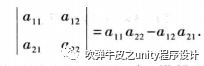也就常说的 ad - bc计算示例有：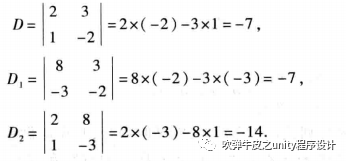多阶行列式的计算方法对于多阶的行列式，小菜知道的有两种方法。降阶法。对角线法。降阶法大学课程中的重点讲解就是这种方法。把一个n阶行列式中的元素aij所在的第i行和第j列划去后，留下来的n-1阶行列式叫做元素aij的余子式,记作Mij。记Aij=(-1)i+jMij，叫做元素aij的代数余子式。例如：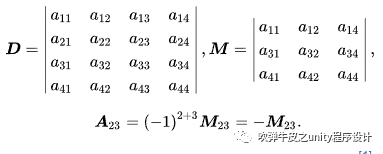给定的矩阵D，在划去a23后得到的余子式为M。元素a23的代数余子式为A23。一个n×n矩阵的行列式等于其任意行(或列)的元素与对应的代数余子式乘积之和。即：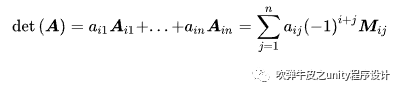由上面公式的引导，故有：1)三阶矩阵行列式以划去第一行为例(划去任意一行或者一列都可以，其运算结果不变)：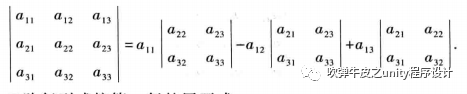可以看出利用降阶法，轻松的将一个三阶矩阵行列式的计算变换成了一个只有二阶矩阵的运算过程。一个简单求解的例子：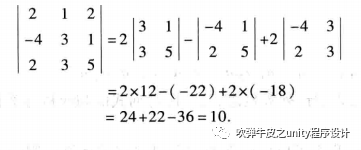2)四阶矩阵行列式利用代数余子式最终都可以将高阶矩阵转换成二阶矩阵来计算。四阶矩阵行列式的求解也需要层层降阶。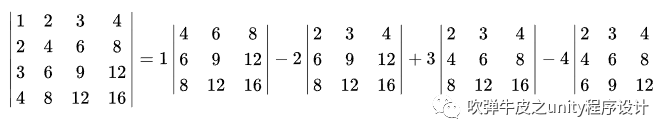对角线法对角线法求解矩阵行列式，顾名思义是根据矩阵式子的正对角线和副对角线之差的运算法则。再回头来看一开始给出的二阶矩阵的计算是不是就是满足对角线法则。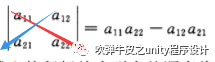1)三阶矩阵行列式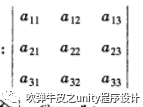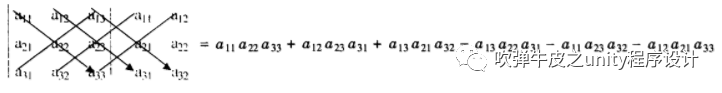用所有正对角线的乘积结果减去所有的副对角线的乘积结果。2)四阶矩阵行列式首先还是来仔细研究下三阶矩阵的行列式值的结构。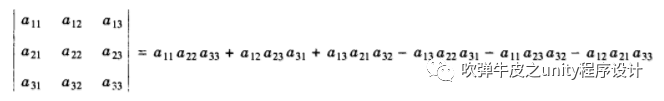首先三阶行列式的值是6项式子的和，其中每一项都是不同行不同列上的3个数的乘积。其次，观察每一项的+ - 符号的决定，小菜定义数aij为行列式的第i行第j列元素，它的第1个足标称为行标，第2个足标称为列标，把每一项的3个元素依行由小到大的舒徐排列起来观察列标排列的特点。在一个排列中如果大数t在小数r的前面，则称此排列有1个逆序。在上述各项列标的排列中，如排列231，2在1前面，有1个逆序；3在1前面，又有1个逆序。因此排列231共有2个逆序即逆序数是2，又如排列123没有逆序即逆序数为0。规定列标排列的逆序数为偶数时，该项取"+",为奇数时，取"-"。因此，a11a22a33,a12a23a31,a13a21a32等3项的符号为"+";而a13a22a31,a11a23a21,a12a21a33的符号为"-"。根据对三阶行列式的分析，小菜进一步探讨利用对角线法则来计算四阶行列式。先将1个四阶行列式写成3种不同形式，分别求出他们的值。(1)第1种形式。将第1、第2和第3列按顺序添加在原矩阵第4列的后面，其中每列上方标记"+"和"-"分别表示该列按箭头方向4个元素乘积的符号。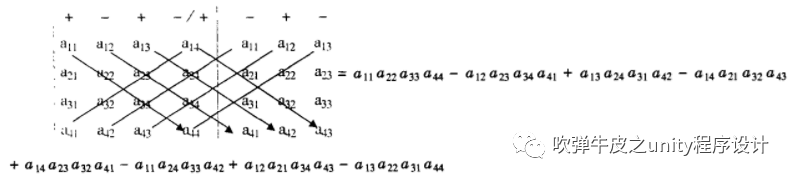(2) 第2种形式。将原四阶行列式的前3列进行1次轮换形成1个新的四阶行列式，再按照第1种形式的方法计算。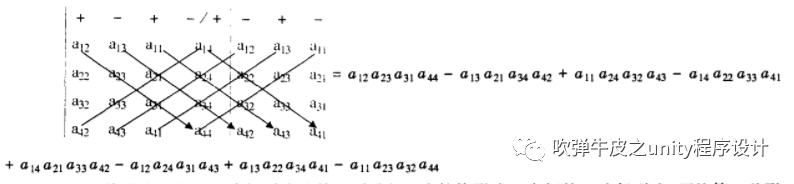(3) 第3种形式。将原四阶行列式的前3列进行2次轮换形成1个新的四阶行列式，再按照第1种形式的方法计算。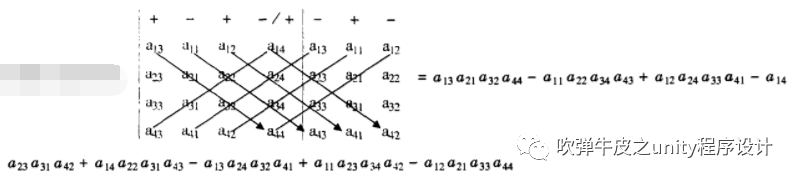最后将这3种形式计算结果求和得到。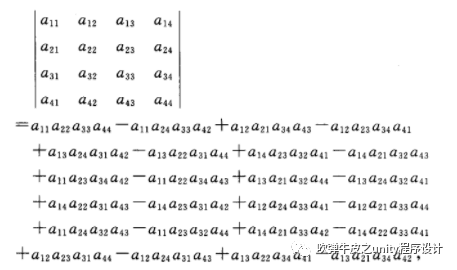最终求得的便是四阶矩阵行列式的最终结果。计算示例：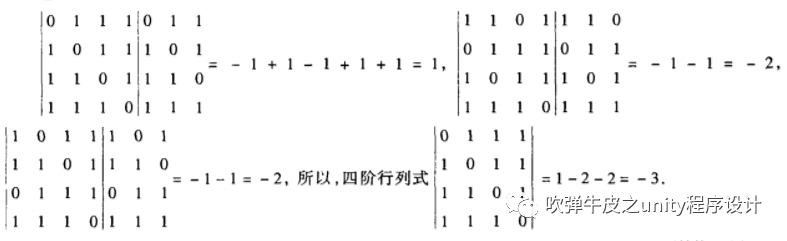编码：理论研究完成了，赶紧将这一成果应用到自己的矩阵类上。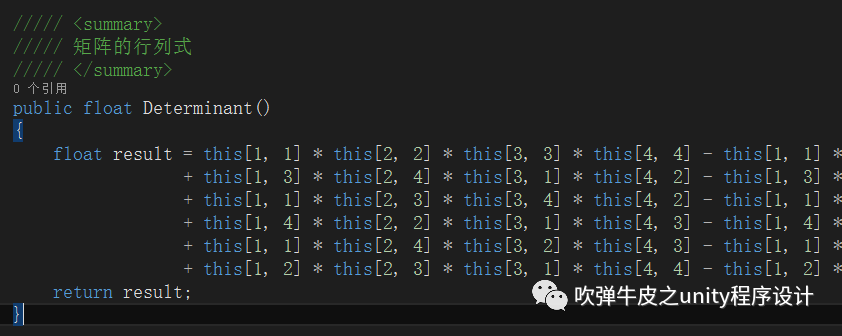行列式的几何意义1)二阶行列式二阶行列式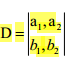的几何意义是xoy平面上以行向量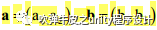为邻边的平行四边形的有向面积。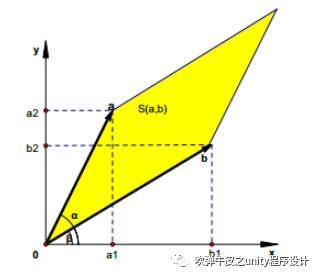1)三阶行列式三阶的行列式是其行向量或列向量所张成的平行六面体的有向体积。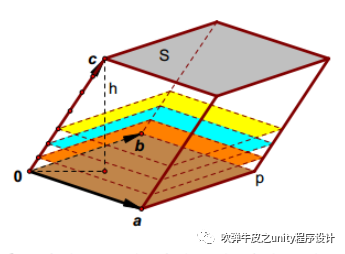谢谢大家的观看阅读，和大家的长期支持！谢谢！

展开全文• 行列式 n*n矩阵（行数列数不同的矩阵是没有行列式的）的行列式用|M|表示，其计算方法是所有主对角线元素乘积之和减去所有反对角线元素乘积之和。2. 余子式与代数余子式 对于矩阵M有m行n列，从矩阵M中移除第i行第j列...
• //行列式计算(5)//01前言(1)今天我们继续讨论特殊行列式的计算思路与方法。今天这组题的行列式的矩阵是一个实对称矩阵, 主对角线是同一个元素, 其余位置是另一元素。该矩阵的行列式有相当好的性质, 需要同学们加以...
• SPOJ -Find The Determinant III ... 参考中还有几个关于行列式的性质。...　计算行列式的基本方法就是把矩阵化成上三角或下三角，然后观察对角线的元素，如果其中有一个元素为0则答案为0，否则行列式的值就是...
• 内容概览：行列式定义行列式计算方法行列式的...A的转置与A的行列式的值相同Cofactor Expansion一个求解矩阵行列式的例子矩阵的特点：除对角线上的值为1，包围对角线的1还有两列1以上矩阵的递归最终都会回到上述三个...
• 将介线性代数中行列式的计算，代数余子式与行列式展开、矩阵的基本运算，矩阵的求逆与伴随矩阵、单位矩阵与对角矩阵特殊矩阵的描述以及矩阵的秩、迹和矩阵方程的等相关问题的求解和相关结论的验证实现方法....
• 行列式的学习一方面要...行列式的计算：1)对于二阶行列式，直接用对角线法；2)对于三阶行列式，可以有对角线法(初学者可以用这个方法)，学了行列式展开后，用展开进行计算即可；3)对于四阶及以上的行列式，用性质化...
• 特征值之积等于矩阵的行列式 假设矩阵的特征值都是整数 例题1 在假设特征值为整数情况下，特征值之积为5，特征值之和为3，那么特征值只可能为5，-1 ,-1 因此直接选出答案。 注意：有经验命题...
• 二阶行列式计算方式：对角线法则三阶行列式计算方式：对角线法则n阶行列式计算排列的逆序数计算n阶行列式简化计算总结行列式的3种表示方法行列式的性质性质1: 行列式与它的转置行列式相等注：行列式中行与列具有同等...
• 这里我们采用按第一列展开，得 二、基本思路• 由于3阶以下的行列式计算可以直接使用对角线法则，因此对于高阶行列式，考虑从降阶角度入手. 降阶具体方法是将行列式按第一列展开，并将代数余子式再按其第一列...
• 行列式的计算方法对角线减副对角线这种计算行列式的方法只适用于三阶及以下的行列式，高阶的行列式的计算需要用后面 的行列式的展开公式 来计算 行列式是——数 矩阵是 ——表格 1.1.2 排列、
• 目录Gauss 消去法矩阵意义矩阵 LU 分解其它三角分解LDU 分解对称正定矩阵带状矩阵分解三对角分解矩阵分解应用1.求解方程组 $Ax=b$ 解2.求矩阵行列式3.求矩阵逆4.求解成组方程组 Gauss 消去法...解方程组
• 整个求解思想就是尽量将矩阵化为对角矩阵，每次取一行，逐渐化简矩阵，在化简过程中，有很多矩阵出现零行或零列，行列式变为0，我们用上述方法对3*3矩阵计算行列式，去掉那些行列式为0项，得到 从上面...
• 不使用行列式的代数体系基础理论十一讲 出版时间：2011年版 内容简介 　《不使用行列式的代数体系基础理论十一讲》的写作宗旨是阐述不使用行列式的代数理论体系的理论基础。与通常意义下的代数体系相比，其特点是不...
• 这里我们采用按第一列展开，得 二、基本思路• 由于3阶以下的行列式计算可以直接使用对角线法则，因此对于高阶行列式，考虑从降阶角度入手. 降阶具体方法是将行列式按第一列展开，并将代数余子式再按其第一列...c语言 计算器 c语言计算器 自己动手写操作系统
• 这一部分内容没有重要内容...三对角线矩阵行列式规律 行列式公式 利用上一讲里拆分性质，可以归纳出这个公式，就比如这样： 最终公式为： detA=∑±a1αa1βa1γ...a1ω，α,β,γ....ω,perm(1...n)det \...线性代数
• 计算机通过主元来计算行列式，但还有另外两种方法，一种是大公式，由项置换矩阵组成；另一种是代数余子式公式。主元乘积为。大公式有项，但只有 5 个非零项。16 来自于对角线上 4 个 2 乘积，其余通过公式我们...
• 计算机通过主元来计算行列式，但还有另外两种方法，一种是大公式，由项置换矩阵组成；另一种是代数余子式公式。主元乘积为。大公式有项，但只有 5 个非零项。16 来自于对角线上 4 个 2 乘积，其余通过公式我们...
• 计算机通过主元来计算行列式，但还有另外两种方法，一种是大公式，由 $$n!$$ 项置换矩阵组成；另一种是代数余子式公式。 主元乘积为 $$2 * \frac{3}{2}* \frac{4}{3}* \frac{5}{4} = 5$$。 大公式有 $$4!=24$$ ...
• ★今日复习专题：行列式综合引言：按照复习计划安排，线性代数“行列式”和“矩阵”两个版块...具体型行列式的计算：主要有两大类型、四大方法，分别是—— 爪型行列式、异爪型行列式、三条对角线行列式； 特殊行列...
• 特征值的乘积等于原矩阵A的行列式的值。 特征多项式的乘积等于矩阵之积。 2、具体例子的求解方法 计算：A的特征值和特征向量。 化简 令x=1，便可得出一个基础解系： 同理当λ2=λ3=0λ_2=λ_3=0λ2​=λ3​=...
• 特征值和特征向量求解方法，可以根据定义， 计算使行列式为零 值，再带入定义求解对应特征向量。只有方阵可以计算特征值。奇异值分解 SVD奇异值分解基本目标是得到矩阵 一个分解 。其中，左奇异向量阵 ，...
• 矩阵秩的全选主元高斯消去法、对称正定矩阵的乔里斯基分解与行列式的求值、矩阵的三角分解 、一般实矩阵的QR分解、一般实矩阵的奇异值分解 、求广义逆的奇异值分解法、约化对称矩阵为对称三对角阵的豪斯荷尔德变换...
• 对于高斯消元法，我们可以直观感觉一下高斯消元操作步骤，将下三角元素全都变成0，这时候对角线上元素就是主元，主元不能为零，如果主元中出现了零，那么该矩阵的行列式就等于0（行列式等于主元之积）。...
• 第1章 矩阵的初等性质 1.1 矩阵及其初等运算 1.1.1 矩阵和向量 习 题 1.1 1.1.2 矩阵的分块乘法与初等变换 习 题 1.2 1.2 矩阵的行列式矩阵的秩 1.2.1 行列式及其性质 习 题 1.3 1.2.2 矩阵的秩及其性质 习 题 1.4...